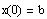b) Finding a particular solution satisfying the initial condition:
Display the general solution below
Define the general solution. Use c1, c2 and c3 ( do not use subscripts).
Define and compute the third solution.
Define and compute the second solution.
Define and compute the first solution. Use the symbolic equal.
State the condition on v1 for the method to work.
Define v1 using v2 and use the symbolic equal to display the result.
State the condition on v2 for the method to work.
Define v2 using v3 and use the symbolic equal (the arrow) to display the result.
Display v3.
Define the components of v3. Use subscripts.
Let
Compute the rref of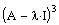So, the eigenvalue is defective with defect 2 and therefore, we find v3 by solving the system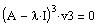for v3
Based on the rref of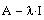, state how many linearly independent eigenvectors can be generated by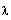. Why?
Compute the rref ofTo find the eigenvector associated with, we solve the homogeneous system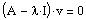for v. This can be achieved by computing the reduced row echelon form of the matrix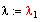Since we only have one eigenvalue we can simplify the computation by assigning the value of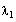toCompute the eigenvalues of A by solving the characteristic equation forand assigning the vector result to.
Write your observation about relationship between this char. eqn and the char. eqn of the scalar differential equation in part A.
Compute the characteristic equation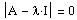.
Define I as the 3 x 3 identity.
Set the origin to 1
a) finding the general solution
Solution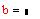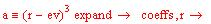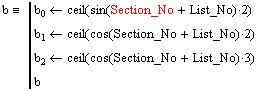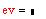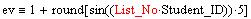End of Part B

End of Project

End of Semester

End of Sufferings
Compare and find the relationship between the solution of the scalar equation and the solution of the system. Write your findings below: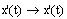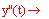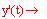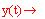The solution of the scalar equation and its derivatives and the solution of the system are displayed below for your convenience.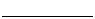If the two side do not match, it means you have made a mistake somewhere in your calculations.
Compute the RHS and compare it with the LHS. Use the simplify command.
Define the RHS as A x(t)
Compute the LHS below. Use the simplify command.
Define the LHS as x'(t)
Define the components of the derivative of the solution as a 3 x 1 vector and name it x'(t).
To verify your solution, you need to compare the left-hand side x'(t) with the right-hand side Ax(t)
Define the general solution (using c1, c2 and c3) one more time and use the symbolic equal to compute the particular solution. Use the simplify command
Define c1, c2 and c3 using subscripts of s (remember the subscript starts at 1)
Use the solve command to find c1, c2 and c3 and define the result as s (see step (b3) in part A).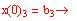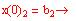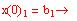To find a particular solution, we need to find the values of c1, c2 and c3 by solving the nonhomogeneous system. This is done by equating corresponding components of the general solution evaluated at 0 and the components of the initial vector b. That iswhere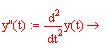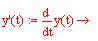1. Compute the first and second derivative of the solution and define them as y'(t) and y''(t), respectively:
b) To find a particular solution satisfying the given initial condition, we perform the following steps:
Note: Here we have a root with multiplicity 3 and we can use any element of the vector r to write the solution.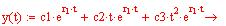3. Use the roots (elements of the vector r) to write the general solution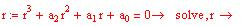2. Use the solve command to find the roots of the characteristic equation and assign the result to r.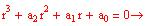1. Use the coefficients of the differential equation to write the characteristic equation in terms of the variable r
a) To find the general solution we do
Solution: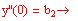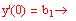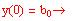a) Find the general solution

b) Find a particular solution satisfying the initial conditions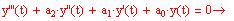Given the homogeneous scalar differential equation:
Part A
This part of the project will be done for you for comparison with the results to be obtained by you in part B.
Start of Project
Instructions

1. Carefully fill out the personal information at the top of this worksheet.

2. Before you start working on the worksheet, use the "save as" command to save the
worksheet as follows:
SxxLyyP2 .mcd
where, xx is your section number

3. T o facilitate the grading of the project, be as neat as possible and place your input in the space provided for you.

4. Unless absolutely necessary, do not add or delete lines into the document. Enough room is given for writing your answers.

5. If for some reason the screen display gets missed up, press Ctrl+R to refresh the screen.

7. You should submit your worksheet in two forms:
a) Softcopy through e-mail
e-mail your worksheet named as explained in part(8) to
afarahat@kfupm.edu.sa
Do not send a zipped file.
b) Hardcopy (Print out) to be submitted on or before Wednesday June 2, 2004.

8. Collaboration is permitted but copying in punishable by an F grade.
Objectives

Solve a homogeneous 3rd order scalar differential equation (the characteristic equation is designed to have a root with multiplicity 3).

Find a particular solution satisfying given initial conditions.

Convert the scalar equation into a system of 3 first-order differential equations.

Solve the system using the eigenvalue-eigenvector method.

Find a particular solution satisfying initial conditions that are equivalent to the conditions of the scalar equation.

Verify the solution.

Compare and find the relationship between the components of the particular solution of the system and the particular solution of the scalar equation and its first and second derivatives
NOTE:
An individual problem will be generated for you using your section number, your list number and your student ID number. Therefore, Section_No, List_No, and Student_ID are defined above as global variables using the global equal " ". Use the placeholder to enter the numbers.

If you have any question or need help, e-mail me at afarahat@kfupm.edu.sa or call my mobile 050-384-7098. Good luck.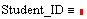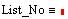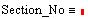Name:
Computer Project Two
May 22, 2004
Math 260-032(A. Farhat)
wherea) Find the general solution

b) Find a particular solution satisfying the initial conditions
where A is (Define your coefficient matrix here) ==>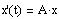So, given the system of differential equations
On a piece of paper, convert the differential equation in part A to a first-order system of the form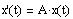and obtain the coefficient matrix A.
A good source to use for help can be found on the WebCT (this hyperlink will take you to the logon page of WebCT). Look for the file "7.5 P25-m3d2.htm" under "Lecture Notes" on the webCT page of the course. or my webpage (this will take you directly to the file).
In this part, as stated in the objectives, you will be asked to convert the scalar differential equation, solved in part A, to a system of first order differential equations. Then, solve the system using the eigenvalue-eigenvector method and compare the two solutions. You will also be asked to find the relationship between components of the system solution and the solution of the scalar equation and its derivatives.
Part B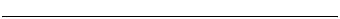value resetting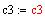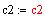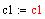End of part AFor a reason to be found later, we also compute the derivatives of the particular solution4. Substitute the computed values of c1, c2, and c3 into the solution to find a particular solution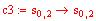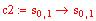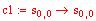Note: The solution is stored in a row vector named s. Therefore, we can assign the values to c1, c2 and c3 as follows: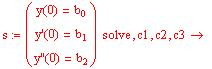3. Use the solve command to find c1, c2 and c32. Evaluate the solution y(t) and its derivatives y'(t) and y''(t) at t = 0 and set them equal to the initial conditions. This will give three equations in the three unknowns c1, c2 and c3 as computed below: